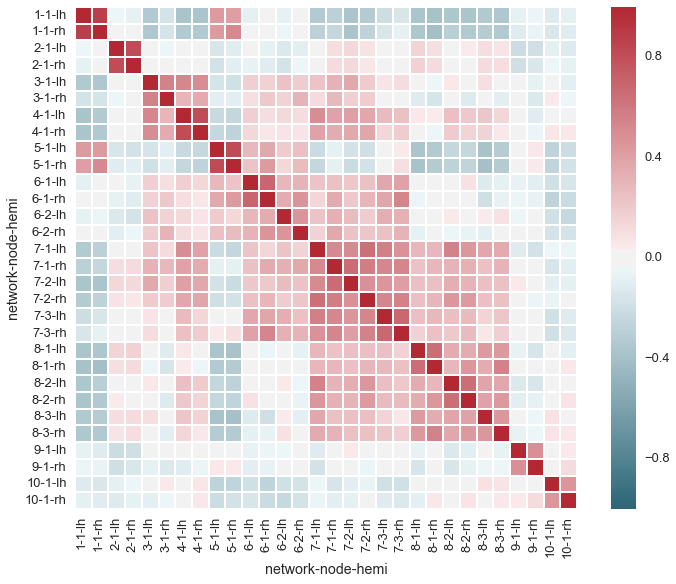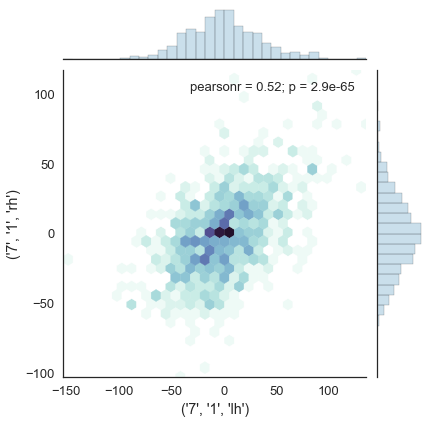# Simple demonstration of interactive color palette widgets¶

This demos a few features that are currently in the development version of seaborn and will soon be in the 0.5 release.

In :
%matplotlib inline

In :
import seaborn as sns

In :
sns.set_context("talk", rc={"figure.figsize": (12, 9)})


Load the intrinsic cortical connecitivty data and compute a correlation matrix

In :
networks = sns.load_dataset("brain_networks", header=[0, 1, 2], index_col=0)
corrmap = networks.iloc[:, :30].corr()


Load a widget to interactively choose a diverging colormap (good for correlation data)

In :
cmap = sns.choose_diverging_palette(as_cmap=True)Plot the correlation matrix as a heatmap

In :
sns.heatmap(corrmap, square=True, linewidths=1, cmap=cmap);Now you can go back and tweak the parameters of the colormap (without rerunning that cell), then rerun the cell with the headmap to see the new colormap in action.

Let's try a different visualization and a correspondingly different colormap.

In :
sns.set_style("white")

In :
cmap = sns.choose_cubehelix_palette(as_cmap=True)In :
sns.jointplot(networks.loc[:, ("7", "1", "lh")],
networks.loc[:, ("7", "1", "rh")],
kind="hex", color=cmap(.4),
joint_kws=dict(cmap=(cmap)));

Out:
<seaborn.axisgrid.JointGrid at 0x1288a57d0>In [ ]: